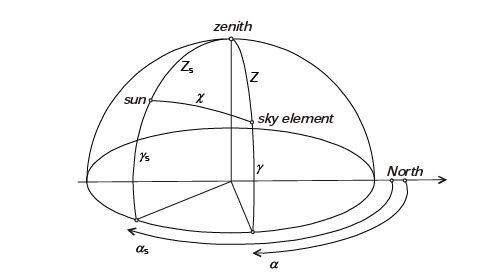# Sky Patch Position _ Sky Luminance Distribution Calculation

Dear All,

Thank you for your great contribution in the field.

I am in a stage of validation of my model using a solar simulator. I need to define the position of a sky patch/element at a specific hour. According to ISO 15469:2004,
"The position of an arbitrary sky element is defined by its zenith angle, Z, and by the azimuth difference between the element and the sun, [α - αs]. If Zs is the zenith angle of the sun, the angular distance between the element and the sun is:

x=arccos⁡(cosZscosZ+sinZssinZ*cos⁡[ α-αs]By using LadyBug_SunPath component we can get the azimuth and the altitude/elevation of the sun. And by LadyBug_SkyDome component we can visualize the sky patches for certain hour, however, I could not find a way to get the azimuth or the altitude of the related sky patch for that hour so I can define the sky position by the above equation.

How can I find either the azimuth or the altitude of the sky patch, theoretically by calculations, please?

Thank you,
Fawzan

Hi Fawzan,

the sky patch position is invariant, it is the coefficients
corresponding to the sky patches that get scaled according to the hourly
average luminances.

Do you mean the patch that represents the direct sun at a given hour? In
that case, you can compute the vector pointing at the sun from the known
azimuth and elevation angles at a given time, and get the patch number
for that vector using the .cal-file that describes your sky subdivision
(Tregenza, Reinhart, …). For a Tregenza sky, you could e.g. use
tregenza.cal with rcal. As an example. With a sun at elevation 45° South
would give a vector (pointing away from the sun) i=(0,.71,-.71), so e.g.

echo “0 -.71 .71” | rcalc -f tregenza.cal -e ‘Dx=\$1; Dy=\$2; Dz=\$3; \$1=tbin’

would give you path number 96. Just be aware that the most CBDM
workflows (and the tools coming with Radiance) distribute the direct sun
flux over n nearest patches.

Cheers, Lars.

Hi Lars,